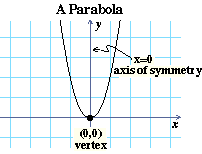# Investigating \$y=(x-h)^2+k\$

Remember that \$a^2\$ means “\$2\$ \$a\$’s multiplied together” (for example, \$(-2)^2=(-2)(-2)=4\$). The equation \$y=x^2\$ is called a quadratic equation because the highest power of a variable is \$x^2\$, whose exponent is \$2\$. In this lesson you will study this and other similar quadratic equations.

If \$y=x^2\$, what will the value of \$y\$ be if \$x=3\$? Enter this value in the \$y\$ column corresponding to \$x=3\$ in the table to the right. Repeat this for all of the other \$x\$ values in the table, using the equation \$y=x^2\$.
\$x\$\$y\$

Notice that the points from the table above are plotted on the grid to the left. Click to graph the equation \$y=x^2\$ on the same grid.

 If you multiply a positive number by itself, is the result positive or negative?
 If you multiply a negative number by itself, is the result positive or negative?
 Can you find any value for \$x\$ so that when you plug it into \$y=x^2\$ you get a negative number?
 What is the smallest possible value \$y\$ can have?
 Is there a largest possible value \$y\$ can have?
 Look at the symmetry of the graph. You should be able to fold the grid along a line so that the points on one side match the points on the other side. What is the line along which you can fold the grid? The -axis
 At which point does the graph cross that line?
 Does the graph ever go below the \$x\$-axis?
 What is the lowest point \$(x,y)\$ on the graph?Parabola: The shape of the graph (shown above) of \$y=x^2\$ is called a parabola. Axis of Symmetry: In this case, the line \$x=0\$ is called the axis of symmetry of the parabola, since you can fold the grid along this line to make the two halves of the parabola match. Vertex: The point where a parabola crosses its axis of symmetry is called the vertex of the parabola.

## Equations of the form \$y=x^2+k\$

\$y=x^2+2\$ is also a quadratic equation because the highest power of \$x\$ is \$x^2\$. In this section you will see how equations like \$y=x^2+2\$ compare to \$y_1=x^2\$.

Complete this table of values for \$y=x^2+2\$. The table has been completed for \$y_1=x^2\$.
\$x\$\$y_1\$\$y\$
 When \$x=3\$, what is the difference between \$y\$ and \$y_1\$? (\$y-y_1\$)
 For any \$x\$ value, what is the difference between \$y\$ and \$y_1\$?
 Notice that the \$y_1=x^2\$ column of the table has symmetry across the \$x=0\$ row (that is, the \$x=1\$ and \$x=-1\$ rows are the same, as are the \$x=2\$ and \$x=-2\$ rows, and so on). Across which \$x\$ value does the \$y=x^2+2\$ column have symmetry?
 Do the blue points seem to be symmetrical across this line on the grid?

Click to graph the two equations \$y_1=x^2\$ and \$y=x^2+2\$.

 Do they have the same axis of symmetry, or different axes of symmetry?
 What is the vertex of the blue graph (the point where it crosses the axis of symmetry)?
 Do the two graphs have the same vertex, or different vertices?

The equation \$y=x^2+k\$ is graphed on the grid to the left. Click on the slider for \$k\$ and move it. As you change \$k\$, the equation for the graph is written below the grid.

 As you change the value of \$k\$ in \$y=x^2+k\$, does its graph stay the same shape or change shape?
 As \$k\$ increases from 0 to 5, does the graph of \$y=x^2+k\$ move up, down, left, or right?
Where are the vertex and axis of symmetry of \$y=x^2+2\$?
 Vertex: Axis:
Where are the vertex and axis of symmetry of \$y=x^2+4\$?
 Vertex: Axis:
 What is the vertex of \$y=x^2+20\$?

## Equations of the form \$y=(x-h)^2\$

\$y=(x-2)^2\$ is also a quadratic equation, even though the \$x^2\$ term is hidden in the way the equation is written. In this section you will learn about equations like \$y=(x-2)^2\$.

Complete the table of values for \$y=(x-2)^2\$.
\$x\$\$y_1\$\$y\$
 Looking at the table, you can see that \$x=0\$ is the axis of symmetry of \$y_1=x^2\$. What is the axis of symmetry of \$y=(x-2)^2\$?
 How many rows do you need to move the \$y_1\$ column up to make it match the \$y\$ column? (If you need to move it down, your answer should be negative.)

Click to graph the two equations \$y_1=x^2\$ and \$y=(x-2)^2\$.

 Are the two graphs the same shape or different shapes?
 Is the graph of \$y=(x-2)^2\$ generally above the graph of \$y_1=x^2\$, below that graph, to the right of that graph, or to the left of that graph?
 What is the vertex of \$y=(x-2)^2\$?

Click on the slider for \$h\$ and move it to change \$h\$ in the equation \$y=(x-h)^2\$.

 As \$h\$ decreases from 0 to \$-5\$, does the graph of \$y=(x-h)^2\$ move up, down, left, or right?

Use the slider to complete the table below.

Equation\$h\$vertexaxis of
symmetry
 What is the axis of symmetry of the graph of \$y=(x+15)^2\$?

## Putting it all together

Here is the graph of the equation \$y=(x-h)^2+k\$. Using the sliders for \$h\$ and \$k\$, change their values and notice the effect on the graph. The equation for the parabola is shown below the grid.

Complete the table below.

Equation\$h\$\$k\$vertexaxis of
symmetry
 Where is the vertex of the parabola \$y=(x-2.2)^2+1.3\$?
 Write the equation for a parabola in the form \$y=(x-h)^2+k\$, if its vertex is at \$(2,3)\$.
 Does changing \$h\$ make the graph of \$y=(x-h)^2+k\$ move up and down, or left and right?
 Does changing \$k\$ make the graph of \$y=(x-h)^2+k\$ move up and down, or left and right?
 What is the vertex of the graph of \$y=(x-h)^2+k\$?
 What is the axis of symmetry of the graph of \$y=(x-h)^2+k\$?
 If \$y=(x-h)^2+k\$, what is the smallest possible value \$y\$ can have?
 Is there a largest possible value \$y\$ can have?